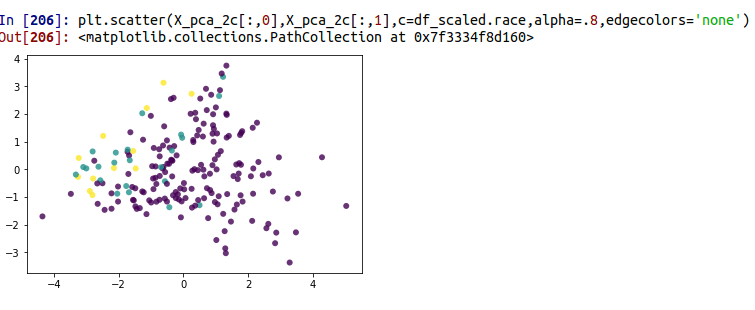# Principal Component Analysis in Python

Principal component analysis is a form of dimension reduction commonly used in statistics. By dimension reduction, it is meant to reduce the number of variables without losing too much overall information. This has the practical application of speeding up computational times if you want to run other forms of analysis such as regression but with fewer variables.Another application of principal component analysis is for data visualization. Sometimes, you may want to reduce many variables to two in order to see subgroups in the data.

Keep in mind that in either situation PCA works better when there are high correlations among the variables. The explanation is complex but has to do with the rotation of the data which helps to separate the overlapping variance.

Prepare the Data

We will be using the pneumon dataset from the pydataset module. We want to try and explain the variance with fewer variables than in the dataset. Below is some initial code.

```import pandas as pd
from sklearn.decomposition import PCA
from pydataset import data
from sklearn.preprocessing import StandardScaler
import matplotlib.pyplot as plt```

Next, we will set up our dataframe. We will only take the first 200 examples from the dataset. If we take all (over 3000 examples), the visualization will be a giant blob of dotes that cannot be interpreted. We will also drop in missing values. Below is the code

```df = data('pneumon')
df=df.dropna()
df=df.iloc[0:199,]```

When doing a PCA, it is important to scale the data because PCA is sensitive to this. The result of the scaling process is an array. This is a problem because the PCA function needs a dataframe. This means we have to convert the array to a dataframe. When this happens you also have to rename the columns in the new dataframe. All this is done in the code below.

```scaler = StandardScaler() #instance
df_scaled = scaler.fit_transform(df) #scaled the data
df_scaled=df_scaled.rename(index=str, columns={0: "chldage", 1: "hospital",2:"mthage",3:"urban",4:"alcohol",5:"smoke",6:"region",7:"poverty",8:"bweight",9:"race",10:"education",11:"nsibs",12:"wmonth",13:"sfmonth",14:"agepn"}) # renamed columns```

Analysis

We are now ready to do our analysis. We first use the PCA function to indicate how many components we want. For our first example, we will have two components. Next, you use the .fit_transform function to fit the model. Below is the code.

```pca_2c=PCA(n_components=2)
X_pca_2c=pca_2c.fit_transform(df_scaled)```

Now we can see the variance explained by component and the sum

```pca_2c.explained_variance_ratio_
Out: array([0.18201588, 0.12022734])

pca_2c.explained_variance_ratio_.sum()
Out: 0.30224321247148167```

In the first line of code, we can see that the first component explained 18% of the variance and the second explained 12%. This leads to a total of about 30%. Below is a visual of our 2 component model the color represents the race of the respondent. The three different colors represent three different races.Our two components do a reasonable separating the data. Below is the code for making four components. We can not graph four components since our graph can only handle two but you will see that as we increase the components we also increase the variance explained.

```pca_4c=PCA(n_components=4)

X_pca_4c=pca_4c.fit_transform(df_scaled)

pca_4c.explained_variance_ratio_
Out: array([0.18201588, 0.12022734, 0.09290502, 0.08945079])

pca_4c.explained_variance_ratio_.sum()
Out: 0.4845990164486457```

With four components we now have almost 50% of the variance explained.

Conclusion

PCA is for summarising and reducing the number of variables used in an analysis or for the purposes of data visualization. Once this process is complete you can use the results to do further analysis if you desire.